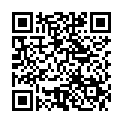## Snake - KS2 Maths Game

Use the arrow keys on a computer or swipe on a tablet to guide the snake to the correct answers.

There are more than 100 different levels to play. See below for a full list of levels.Scan to open this game on a mobile device. Right-click to copy and paste it onto a homework sheet.

### Game Objectives

Multiplication:

 1. Recall and use doubles of all numbers to 10 2. 2 times table 3. 5 times table 4. 10 times table 5. Derive and use doubles of simple two-digit numbers 6. 3 times table 7. 4 times table 8. 8 times table 9. Double numbers up to 100 10. Doubles of multiples of 50 up to 500 11. 6 times table 12. 7 times table 13. 9 times table 14. 11 times table 15. 12 times table

Roman Numerals:

 1. Read Roman numerals up to 12 2. Read Roman numerals up to 50 3. Read Roman numerals up to 100

 1. Read numbers to 20 in numerals and words 2. Read numbers to 100 in numerals and words 3. Read numbers to 1000 in numerals and words

Fractions of Numbers:

Multiples:

 Multiples of 2 Multiples of 3 Multiples of 4 Multiples of 5 Multiples of 6 Multiples of 7 Multiples of 8 Multiples of 9 Multiples of 10 Multiples of 11 Multiples of 12

Factors, Square and Prime Numbers:

 1. Factors of numbers up to 100 2. Prime numbers 3.Square numbers

Simplify Fractions:

 1. Fractions in their simplest form (unit fractions) 2. Fractions in their simplest form (multi-part fractions)

Multiply Fractions:

 1. Multiply unit fractions 2. Multiply multi-part fractions

Convert Improper Fractions to Mixed Numbers

Convert between Fractions and Decimals:

 1. Convert unit fractions to decimals 2. Convert multi-part fractions to decimals 3. Convert decimals to unit fractions 4. Convert decimals to multi-part fractions

Convert between Fractions and Percentages:

 1. Convert unit fractions to percentages 2. Convert multi-part fractions to percentages 3. Convert percentages to unit fractions 4. Convert percentages to multi-part fractions

Rounding Numbers:

 1. Round numbers up to 100 to the nearest 10 2. Round numbers up to 1000 to the nearest 10 3. Round decimals with 1 decimal place to the nearest whole number 4. Round decimals with 2 decimal place to the nearest whole number, or decimal

Division:

 1. Halve numbers up to 20 2. Division facts for the 2 times table 3. Division facts for the 5 times table 4. Division facts for the 10 times table 5. Derive and use halves of simple 2-digit numbers 6. Division facts for the 3 times table 7. Division facts for the 4 times table 8. Division facts for the 8 times table 9. Halve numbers up to 200 10. Division facts for the 6 times table 11. Division facts for the 7 times table 12. Division facts for the 9 times table 13. Division facts for the 11 times table 14. Division facts for the 12 times table 15. Halve decimals (1 d.p.) 16. Halve decimals (2 d.p.)

Percentages of Numbers

 1. Find 10%, 25% and 50% of numbers 2. Find multiples of 10% of numbers 3. Find multiples of 5% of numbers 4. Find multiples of 10% of numbers(inc. decimal answers)